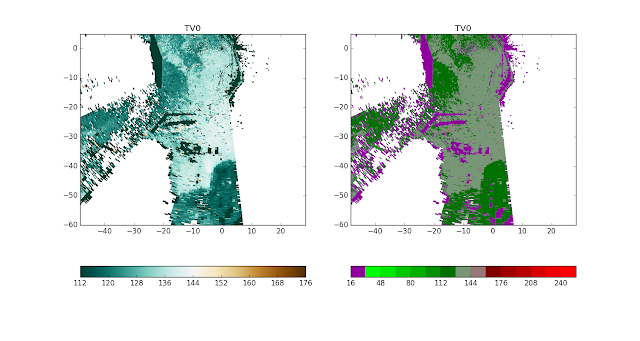This page demonstrates Python tips and tricks that I use in my everyday programming as an atmospheric science graduate student.
-Brian Blaylock

## Thursday, July 7, 2016

### Unidata Python Training

Some good resources to learn Python

https://unidata.github.io/online-python-training/

## Friday, July 1, 2016

### Plotting radar data with MetPy, pyproj, Basemap

#### MetPy radar plots

The MetPy python package is really helpful for atmospheric scientists which allows you to plot radar data (specifically Level 3 data) downloaded from the THREDDS server whose files are in the .nids format. How to use MetPy to plot these data is explained on the MetPy docs, and you'll create a plots of reflectivity and radial velocity like this...
There are two things the example doesn't tell you...

1) The data value units are arbitrary. Notice that the values of dbz color scale is the same as the values in the radial velocity scale on the right. What the MetPy example doesn't tell you is that you need to use the map_data instance to convert the data to appropriate units.

f = Level3File(FILE)

# Pull the data out of the file object (this stuff is in 8-bit)

# Turn into an array, then mask
data = f.map_data(datadict['data']) # I'm confident this maps the data to floats with units of m/s
data = ma.array(data)

2) You don't get a sense of where the radar and its observations are located in relation to places, just a distance from the center. You can convert the azimuth and range into a latitude and longitude by using the pyproj module. Then you can use the lon and lat variables to plot the data on a basemap. Given the center latitude, center longitude, azimuth (degrees from north), and the range from center lat/lon, you can calculate the latitude and longitude of a distant point like this...

from pyproj import Geod

# Grab azimuths and calculate a range based on number of gates
rng = np.linspace(0, f.max_range, data.shape[-1] + 1)

# Convert az, range to a lat/lon
g = Geod(ellps='clrk66') # This is the type of ellipse the earth is projected on.
# There are other types of ellipses you can use,
# but the differences will be small
center_lat = np.ones([len(az),len(rng)])*f.lat
center_lon = np.ones([len(az),len(rng)])*f.lon
az2D = np.ones_like(center_lat)*az[:,None]
rng2D = np.ones_like(center_lat)*np.transpose(rng[:,None])*1000
lon,lat,back=g.fwd(center_lon,center_lat,az2D,rng2D)
#...(add code to create map)
m.pcolormesh(lon,lat,data,cmap="BrBG_r",vmax=10,vmin=-10)

You can replace the xlocs and ylocs in the MetPy example with the lon and lat variables, and use those lons/lats to plot on a basemap.
(Note: back_az is the azimuth from the point back to the center location)

#### TDWR plot on Basemap

I like to look at the TDWR base radial velocity scans to look for lake breezes in the Salt Lake Valley. Below is plotted the radial velocity on June 19, 2015 at 00:24 UTC using MetPy without converting to correct units using map_data and has not been plotted on a basemap (two color schemes used).both plots are the same, but the color bar is different.
It is so much nicer to view radar data on a basemap plot, as shown below, so you get a sense of there the observations are in space. You can see a lake breeze boundary in the center of the valley (below center of the image where color changes from dark green to light green). I have also used the map_data instance to covert the data to units of ms-1. Each plot is the same with different color schemes. Left is the Brown/BlueGreen that I like, and right is the NWS Red/Green scheme built into MetPy.The full code to do this is on github:
Plotting TDWR Radar data:
https://github.com/blaylockbk/pyBKB_v2/blob/master/TDWR/plot_TDWR_reflectivity.py
Plotting NEXRAD Level 2 data:

Extra things you can put on the map are a shape file of the roads, and weather observations from the MesoWest API. Let me know if you find this useful :)